# Implement a pulse-density modulator on an FPGA

2016-09-27

Pulse-density modulation (PDM) is an attractive alternative to pulse-width modulation (PWM) in applications where the PWM technique creates unwanted spikes in the signal spectrum. We present the implementation of a pulse-density modulator on an FPGA to control the current of a laser.

We will use a Red Pitaya board which has 4 slow analog outputs. These are simply digital outputs followed by an RC low-pass filter of about 100 kHz frequency cutoff. The digital pin can switch between 0 and 1.8 V. If the pin is switched fast enough, the analog output only see the average voltage. A 10-bit pulse-width modulator running at 250 MHz (4 ns clock period) can take 1024 values between 0 and 1.8 V. At mid-range the digital pin follows a square-wave of frequency 244 kHz (250 MHz / 1024) and 50 % duty cycle. With pulse-density modulation, this translates into a square-wave of frequency 125 MHz, which is much easier to filter.

## Basic principle

A pulse-density modulator can be thought of as a 1-bit DAC that tries to minimize the accumulated quantization error. At each clock cycle, the error is updated and compared with the input signal: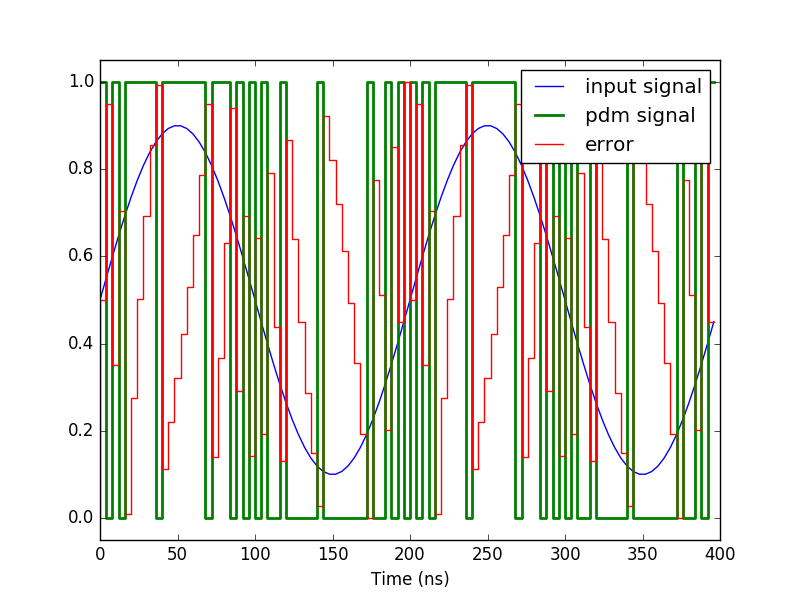Below is the basic PDM algorithm written in Python (full code):

def pdm(x):
n = len(x)
y = np.zeros(n)
error = np.zeros(n+1)
for i in range(n):
y[i] = 1 if x[i] >= error[i] else 0
error[i+1] = y[i] - x[i] + error[i]
return y, error[0:n]


## Hardware implementation

The PDM algorithm is sequential by nature and is thus well adapted for implementation on an FPGA. Although it is possible to perform floating point operations in the FPGA, it is much easier to work with unsigned integer. As we want to implement a pulse-density modulator with 10-bit precision, the input signal din and the error signal can take any integer value between 0 and 1023. Below is the Verilog HDL code used to describe the circuit:

// Pulse density Modulator
timescale 1 ns / 1 ps

module pdm #(parameter NBITS = 10)
(
input wire                      clk,
input wire [NBITS-1:0]          din,
input wire                      rst,
output reg                      dout,
output reg [NBITS-1:0]          error
);

localparam integer MAX = 2**NBITS - 1;
reg [NBITS-1:0] din_reg;
reg [NBITS-1:0] error_0;
reg [NBITS-1:0] error_1;

always @(posedge clk) begin
din_reg <= din;
error_1 <= error + MAX - din_reg;
error_0 <= error - din_reg;
end

always @(posedge clk) begin
if (rst == 1'b1) begin
dout <= 0;
error <= 0;
end
else if (din_reg >= error) begin
dout <= 1;
error <= error_1;
end else begin
dout <= 0;
error <= error_0;
end
end

endmodule


Vivado® translates the hardware description into the following circuit: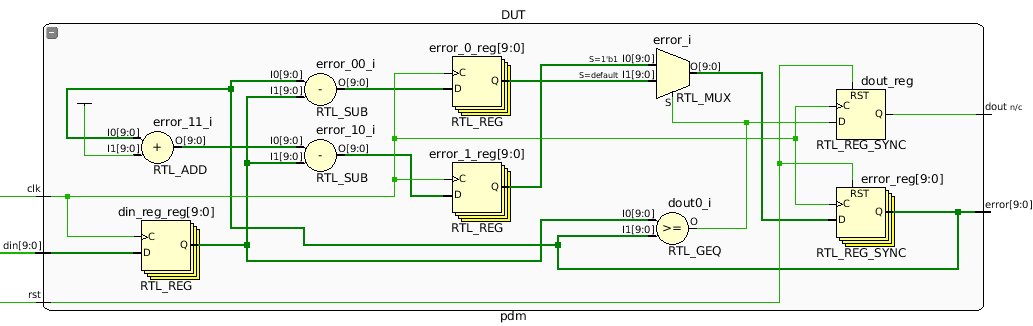## Running the core at 375 MHz

The design above achieves timing closure with a clock frequency of 250 MHz. However, it cannot be pushed at 375 MHz because of the following critical path: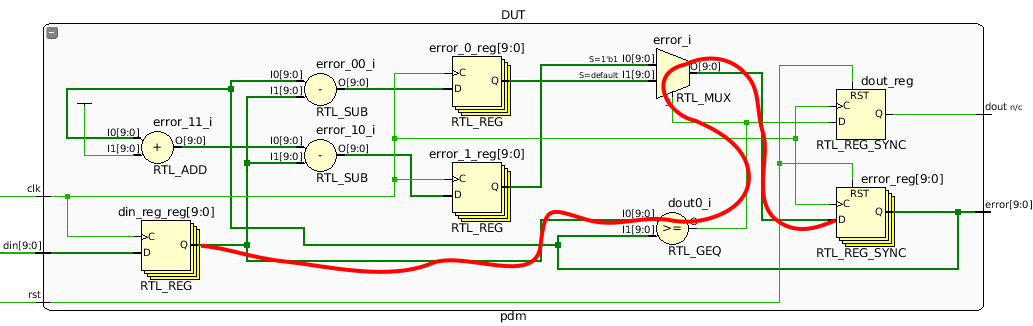The signal has to go through a comparator and a multiplexer in one clock cycle. The problem can be solved by adding a register just after the comparator dout0_i. Fortunately, this can be done without adding any more register since it is already there (dout_reg`). We however have to add a register for the reset signal. The figure below shows the circuit of the final design that achieves timing closure at 375 MHz: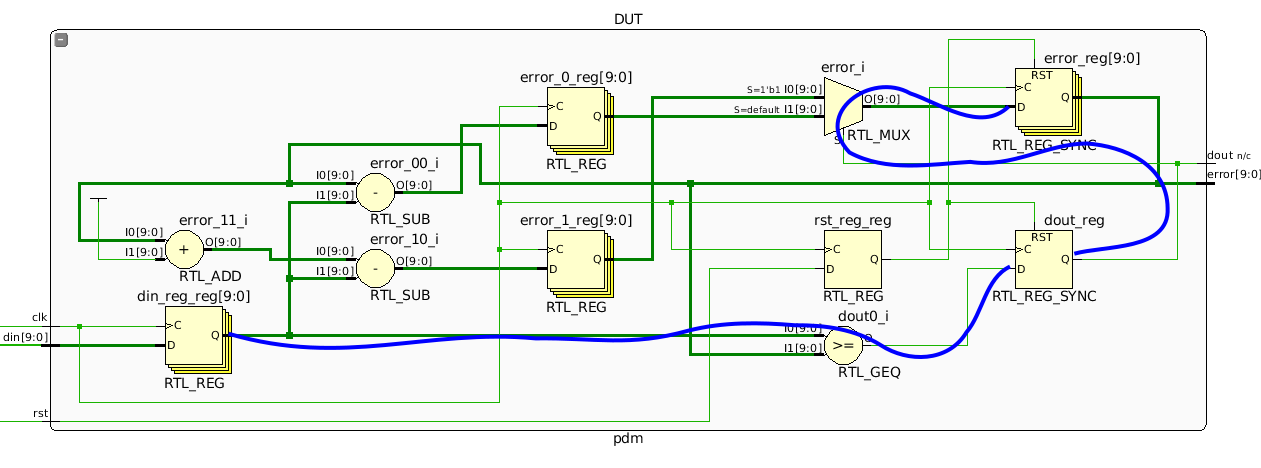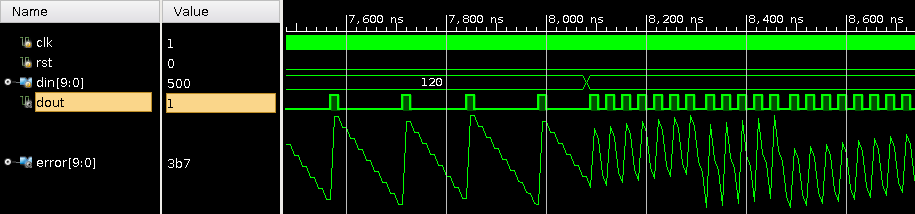### Source code

https://github.com/Koheron/koheron-sdk/tree/master/examples/red-pitaya/laser-controller